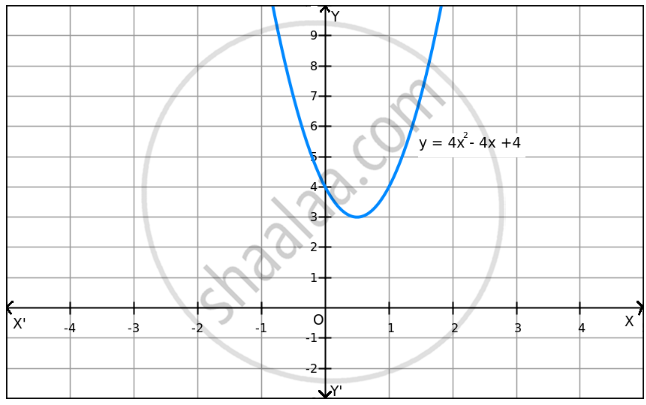Share

# F ( X )=4 X 2 -4 X + 4 on R . - Mathematics

#### Question

f(x) = 4x2 + 4 on R .

#### Solution

Given: f(x) = 4x2 − 4x + 4

$\Rightarrow$ f(x) = (4x2 − 4x + 1)+3

$\Rightarrow$ f(x) = (2x − 1)2 + 3

Now,
(2x − 1)$\geq$ 0 for all x $\in$ R $\in$

$\Rightarrow$ f(x) = (2x − 1)2 + 3 $\geq$ 3 for all x $\in$ R

$\Rightarrow$ f(x) $\geq$ 3 for all x $\in$ RThe minimum value of f is attained when (x − 1) = 0.
(2x − 1) = 0
⇒ x = $\frac{1}{2}$

Thus, the minimum value of f (x) at x = $\frac{1}{2}$ is 3.

Since f(x) can be enlarged, the maximum value does not exist, which is evident in the graph also.

Hence, function f does not have a maximum value .

Is there an error in this question or solution?